Refer to our Texas Go Math Grade 1 Answer Key Pdf to score good marks in the exams. Test yourself by practicing the problems from Texas Go Math Grade 1 Lesson 11.1 Answer Key Model Addition.

Unlock the Problem

Hanna has 4 red flowers in a.
She puts 2 more flowers in the.
How many flowers are in the?
How can you use a model to find out?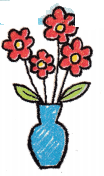What information am I given?
__________ red flowers
__________ more flowers

Plan

What is my plan or strategy?
I can _____________

Solve

Show how you solve the problem.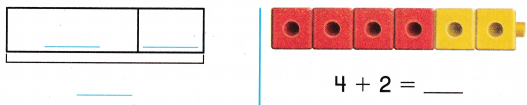Answer: 4 red flowers and 2 yellow flowers in theHOME CONNECTION • Your child con model the concepts of joining, adding to, and putting together. He or she used a model to show the problems and solve.

Try Another Problem

Use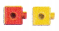to help you. Complete the model and the number sentence.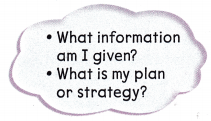Question 1.
There are 7 dogs in the park. Then I more dog joins them. How many dogs are in the park now?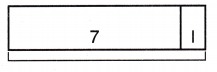7 + 1 = ___________
There are 7 dogs in the park. Then I more dog joins them.
7 + 1 = 8
8

Question 2.
Some birds are sitting in the tree. Four more birds sit in the tree. Then there are 9 birds. How many birds were in the tree before?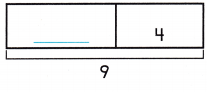_________ + 4 = 9
Some birds are sitting in the tree.
Four more birds sit in the tree.
Then there are 9 birds.
9 – 4 = 5Question 3.
There are horses in the field. Some more horses run to the field. Now there are 10 horses in the field. How many horses ran to the field?4 + _________ = 10
There are horses in the field. Some more horses run to the field.
Now there are 10 horses in the field.
10 – 4 = 6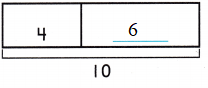Math Talk
Mathematical Processes

Share and Show

Useto help you. Complete the model and the number sentence.

Question 4.
Luis has 12 crayons. 5 of the crayons are red. The rest are blue. How many crayons are blue?5 + ________ = 12
Given,
Luis has 12 crayons. 5 of the crayons are red.
12 – 5 = 7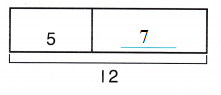Therefore 7 crayons are blue.

Problem Solving

Question 5.
H.O.T. Some ducks are swimming in a pond. 3 more ducks swim in the pond. Then there are 6 ducks in the pond. How many ducks were in the pond before?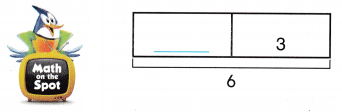__________ + 3 = 6
Some ducks are swimming in a pond.
3 more ducks swim in the pond.
Then there are 6 ducks in the pond.
6 – 3 = 3Thus there were 3 ducks in the pond before.

Question 6.
H.O.T. Multi-Step
_______ bugs are flying. _________ more bugs fly with them. Now there are 10 bugs flying.7 bugs are flying.
3 more bugs fly with them.
Now there are 10 bugs flying.Use. Choose the correct answer.

Question 7.
Use Diagrams Cho sees 3 red flowers. Ki sees 2 yellow flowers. How many flowers are there?(A) 5
(B) 3
(C) 2
Given,
Cho sees 3 red flowers. Ki sees 2 yellow flowers.
3 + 2 = 5
Thus the correct answer is option A.

Question 8.
Ben has 5 books. Kelli has 3 books. Which number sentence shows how many books there are?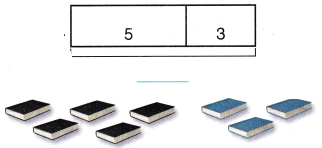(A) 5 + 1 = 6
(B) 5 + 3 = 8
(C) 5 + 2 = 7
Given,
Ben has 5 books. Kelli has 3 books.
5 + 3 = 8
Thus the correct answer is option B.

Question 9.
Multi-Step Some cats sit on a fence. 4 cats join them. Now there are 8 cats on the fence. How many cats were on the fence before?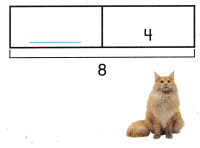(A) 3
(B) 2
(C) 4
Given,
Some cats sit on a fence. 4 cats join them.
Now there are 8 cats on the fence.
8 – 4 = 4
Thus the correct answer is option C.

Question 10.
Texas Test Prep 6 bees are in the hive. 3 more bees fly into the hive. How many bees are in the hive now?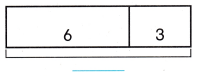(A) 3
(B) 9
(C) 4
Given,
6 bees are in the hive. 3 more bees fly into the hive.
6 + 3 = 9
Thus the correct answer is option B.

TAKE HOME ACTIVITY • Have your child describe each of the ports of a model using the number sentence 7 + 3 = 10.

### Texas Go Math Grade 1 Lesson 11.1 Homework and Practice Answer Key

Complete the model and number sentence.

Question 1.
There are 4 plates on the table. You put 3 more plates on the table. How many plates are on the table now?4 + 3 = ___________
Given,
There are 4 plates on the table. You put 3 more plates on the table.
4 + 3 = 7

Question 2.
8 fish swim in a pond. Some more fish swim into the pond. Now there are 11 fish in the pond. How many fish swam into the pond?8 + _________ = 11
Given,
8 fish swim in a pond. Some more fish swim into the pond.
Now there are 11 fish in the pond.
11 – 8 = 3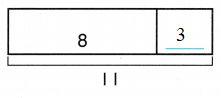Problem Solving

Question 3.
Some bees are flying around a flower. 7 more bees fly with them. Now there are 13 bees flying. How many bees were flying before?________ + 7 = 13
Given,
Some bees are flying around a flower.
7 more bees fly with them.
Now there are 13 bees flying.
13 – 7 = 6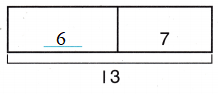Thus there were 6 bees flying before.

Lesson Check

Question 4.
Jerry sees 5 blue cars. Tina sees 6 red cars. How many cars are there?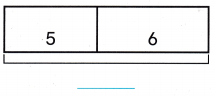(A) 5
(B) 11
(C) 6
5 + 6 = 11
Thus the correct answer is option B.

Question 5.
Cal has 7 pens. Zeb has 2 pens. Which number sentence shows how many pens there are?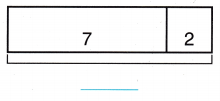(A) 7 + 2 = 9
(B) 7 – 2 = 5
(C) 7 + 1 = 8
Given,
Cal has 7 pens. Zeb has 2 pens.
7 + 2 = 9
Thus the correct answer is option A.

Question 6.
Casey has some shells. Sal gives Casey 4 shells. Now Casey has 6 shells. How many shells did Casey have before?(A) 4
(B) 6
(C) 2
Given,
Sal gives Casey 4 shells. Now Casey has 6 shells.
4 + 2 = 6
Thus the correct answer is option C.

Question 7.
3 squirrels are in a tree. 5 more squirrels join them. How many squirrels are in the tree now?(A) 8
(B) 7
(C) 9